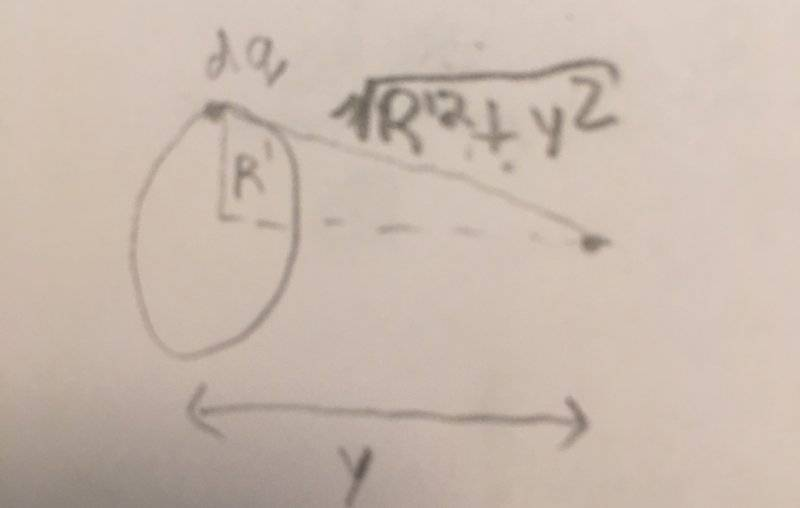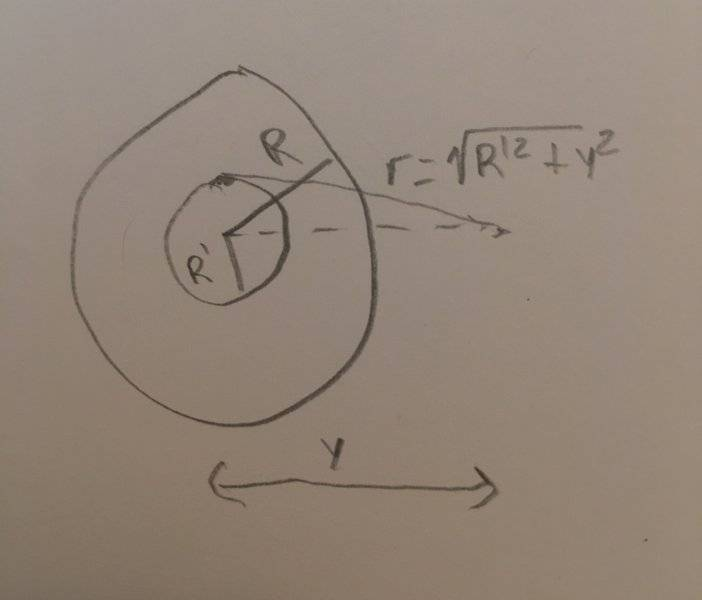# Derivation of electric potential charged disk from ring

## Homework Statement

a)recall that electric potential is a scalar quantity. For a circular ring of radius, R', carrying charge, Q, what is the electric potential at a height,y, above the center of the ring?
b)Use your above answer to determine the electric potential at a height,y, above the center of a uniformly charged disk of radius, R.
c)if y>>R, use the binomial expansion (keeping terms up to and including R2/y2 ) to show that the electric potential now resembles that of a point charge

V=q/(4πε0r)

## The Attempt at a Solution

a)V=q/(4πε0r)
dv=dq/(4πε0)
See ring.jpg attached
V=∫dv=∫dq/(4πε0)=[1/(4πε0r)]∫dq=q/4πε0r=q/4πε0(R'2+y2)1/2

b)See disk.jpg attached
V=kq/(R'2+y2)1/2
dV=kdq/(R'2+y2)1/2

(dq/Q)=(2πR'dr/πR2)→dq=2QR'dr/R2
The limits on the below integral are from 0→R until stated differently
V=∫dV=∫(k2QR'dr)/[R2(R'2+y2)1/2]=(kQ/R2)∫2R'dr/(R'2+y2)1/2
Usub:
U=R'2+y2
dU=2R'dr
dr=dU/2R'
New Limits:
when r=0, U=y2
when r=R, U= R2+y2
after taking integral:
(KQ/R2) [u1/2]evaulated from y2→R2+y2=(KQ/R2)[(R2+y2)-y]

c) This is the part Im confused on... not sure where to start.
I know if y>>R then my above answer turns into
(KQ/R2)[y2-y] but other than that not sure where to go...

Staff Emeritus
Homework Helper
Gold Member

## Homework Statement

a)recall that electric potential is a scalar quantity. For a circular ring of radius, R', carrying charge, Q, what is the electric potential at a height,y, above the center of the ring?
...

V=q/(4πε0r)

## The Attempt at a Solution

a)V=q/(4πε0r)
dv=dq/(4πε0)
See ring.jpg attachedV=∫dv
=∫dq/(4πε0)
=[1/(4πε0r)]∫dq
=q/(4πε0r)
=q/(4πε0(R'2+y2)1/2)
.
This result (Part a) looks good. Remember that the charge, Q is the total charge on the disk, whereas, q, will become the portion of the total charge on a thin ring,

Also, you should enclose in parentheses the whole of any denominators in the above expressions. I added those in RED above.

#### Attachments

•Staff Emeritus
Homework Helper
Gold Member

## Homework Statement

b)Use your above answer to determine the electric potential at a height,y, above the center of a uniformly charged disk of radius, R.

V=q/(4πε0r)

## The Attempt at a Solution

V=q/(4πε0(R'2+y2)1/2)

b)See disk.jpg attachedV=kq/(R'2+y2)1/2
dV=kdq/(R'2+y2)1/2

(dq/Q)=(2πR'dr/πR2)→dq=2QR'dr/R2
The limits on the below integral are from 0→R until stated differently
V=∫dV=∫(k2QR'dr)/[R2(R'2+y2)1/2]=(kQ/R2)∫2R'dr/(R'2+y2)1/2
Any r as in dr you have in this part should be R' and dR'

Usub:
U=R'2+y2
dU=2R'dr
dr=dU/2R'
The u-substitution looks fine, but your integration result doesn't look so good.

Also, after finding the antiderivative (indefinite integral) I find it handy to switch back to the original variable(s) and then substitute the limits of integration: Less chance for mistakes.
New Limits:
when r=0, U=y2
when r=R, U= R2+y2
Before integrating, let's see what the integral is !
∫2R'dr/(R'2+y2)1/2
becomes (without limits for now)
##\displaystyle \int \frac{dU}{\left( U \right)^{1/2}} ##
but you might be better off writing it as:
##\displaystyle \int {U ^{-1/2} \ } dU ##​

You made an error with the integration.

Never mind. the integration looks fine. DUH !

after taking integral:
(KQ/R2) [u1/2]evaulated from y2→R2+y2=(KQ/R2)[(R2+y2)-y]
Well, that last result should be: ##\displaystyle \ V(y)=\frac{2k Q}{R^2}\left( \sqrt{R^2+y^2\,} - y \right) ##

(Fixed a missing factor of 2)

#### Attachments

Last edited:
•Well, that last result should be: V(y)=kQR2(√R2+y2−y)
I re did it also and now Im confusing myself:
Im thinking the final answer should be V(y)=(2KQ/R2)[(√R2+y2-y)]
because evaluating the integral:
∫U-1/2=2U1/2?

Staff Emeritus
Homework Helper
Gold Member
I re did it also and now Im confusing myself:
Im thinking the final answer should be V(y)=(2KQ/R2)[(√R2+y2-y)]
because evaluating the integral:
∫U-1/2=2U1/2?
After posting (#3) I did a couple of quick edits.

A couple of mathematical pointers.

Writing ∫U−1/2 without the dU is a mistake. But, yes, there should be that factor of 2 in the result. (I'll fix my post.)

As for the formula you have for V(y), make sure that the scope of the radical, √ , is clear. Parentheses can help, but it be even clearer to write that with a rational exponent as ( ( R2 + y2)−1/2 − y )

•After posting (#3) I did a couple of quick edits.

A couple of mathematical pointers.

Writing ∫U−1/2 without the dU is a mistake. But, yes, there should be that factor of 2 in the result. (I'll fix my post.)

As for the formula you have for V(y), make sure that the scope of the radical, √ , is clear. Parentheses can help, but it be even clearer to write that with a rational exponent as ( ( R2 + y2)−1/2 − y )
appreciate the pointers. Thank you!
Any suggestions on the last part of the problem?

Staff Emeritus
Homework Helper
Gold Member

## Homework Statement

c)if y>>R, use the binomial expansion (keeping terms up to and including R2/y2 ) to show that the electric potential now resembles that of a point charge

V=q/(4πε0r)

## The Attempt at a Solution

c) This is the part Im confused on... not sure where to start.
I know if y>>R then my above answer turns into
(KQ/R2)[y2-y] but other than that not sure where to go...
Of course we know that last statement is wrong. The potential obtained above is: ## \displaystyle \ V(y)=\frac{2k Q}{R^2}\left( \sqrt{R^2+y^2\,} - y \right) ##
The first thing to do is to manipulate the portion that depends on ##\ y\ ## to to have terms with ##\ R/y \ ##.

A reasonably clean way to do this is to define a new (temporary) variable, ##\ u\,.\ ## Let ##\displaystyle \ u = R/y\,,\ \text{ so that } \ R = u\cdot y \,.\ ##
Notice that if y >> R, then 1 >> u.

You should get ## \displaystyle \ \left( \sqrt{R^2+y^2\,} - y \right) = \left( \sqrt{u^2y^2+y^2\,} - y \right) \,.##

Now, factor out ##\ y \ ## from the expression on the right side of the equation.

"Binomial expansion of what?", you may be asking?

Look at Wikipedia: Newton's generalized binomial theorem .

## \displaystyle \sqrt {x+1} = 1+{\frac {1}{2}}x-{\frac {1}{8}}x^{2}+{\frac {1}{16}}x^{3}-{\frac {5}{128}}x^{4}+{\frac {7}{256}}x^{5}-\cdots ##

Plug in ##\ u^2 \ ## for ##\ x\ ## and see what you get.

Last edited:
•